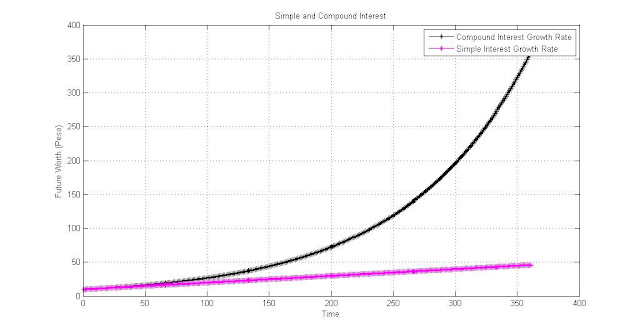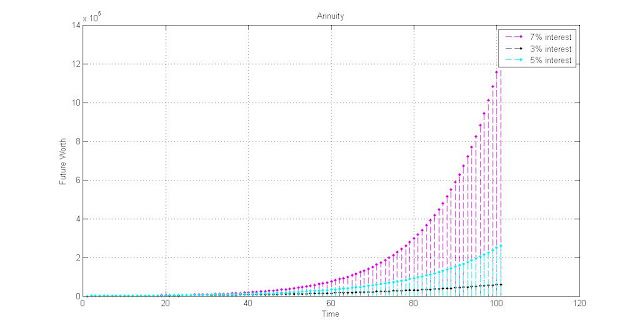Interest

Interest, is formally defined as the price paid for borrowing money or payment received for lending money, usually a percentage of the amount borrowed. My vocabulary defines it as the only reason why a sane person would allow someone else to touch his/her money (expenses for necessities and luxuries aside of course). Without interest, the global economy won't function. Companies won't be able to sell their stocks, no investments will occur, no one would be able to borrow money because no one is INTERESTed to lend their money, etc. In this article, I'll freshen up on the very basic principles that govern interest, and then introduce a conceptual analysis for each.

Before we start with simple interest, let us first discuss an even simpler topic that I believe is taken too much for granted that some errors in academic exams are effectuated by such. Interest is the price paid for borrowing money, but it can be a payment that is paid at a constant value, or a payment that is increasing relative to the original borrowed money. For payments that are paid at constant value, time is simple. But for payments that are paid at an increasing value relative to the original borrowed money, time must be analyzed with more care. The dimension of time needs to be analyzed meticulously or else errors will result. It is helpful to think of time as a variable of interest, not just of the original amount of money involved, and will be proved by theory in the latter discussions.

Simple Interest

I=Pin

In a class discussion, our professor mentioned an easy mnemonics to remember this formula. A Tagalog term for teeth which is "ngipin" that sounds like ipin. But what does it exactly mean? Interest (I) is a function of the present amount of money multiplied by the interest rate (i) in days multiplied by the number of days (n). The higher the present amount, the bigger the interest. The higher the interest, the bigger the interest. The longer the period, the bigger the interest. And the interest gets bigger linearly. For anyone who knows the word linear, they also know that anything accompanying it requires very simple analysis. Hence the name "simple interest" I guess.

Here is a sample figure of the amount of interest you get for a 10 peso and 20 peso, 10 day investment at different rates of simple interest.From the figure above, you can observe that the slope can be taken by dividing the present amount by the number of days. 10 pesos/10 days = slope of 1. 20 pesos/10 days = slope of 2. Notice that higher slopes yield higher returns. Thus, a better slope or a better ratio between the present amount and number of days will yield better returns for simple interest. Take note too that no matter what the values, the output graph is always a line, which is why the equation is called a linear equation.

Of course, if you want to know the future worth of the investment, simply add the interest by the present worth.

F=P+I

Algebraic manipulation can yield other forms of the above equation such as F=P*(1+i*n), P=F*(1+i*n), etc.

Simple interest can be divided into 2 categories when it comes to dimension of time, that is, ordinary simple interest and exact simple interest. Ordinary simple interest is based on a calendar where each month is 30 days long and a year is (30x12) 360 days long whether the year is a leap year or not. Exact simple interest is by its name, more exact when it comes to time. The number of days in a month are based on the actual number, for example, February would be considered to have 28 days and for May, 31 days. Leap years are also considered so if the year is divisible by 4, that year has 366 days in it except for the usual 365.

Sample Problem:

Joseph wants to invest for a certification exam that costs 18000 pesos. He wants to take the exam in 1 year. Right now, he has 10000 pesos. What should be the interest rate for an investment that earns ordinary simple interest?

Solution:

F=P*(1+i*n)

(1+i*n)=F/P

i=((F/P)-1)/n

i=((18000/10000)-1)/360

i=0.002222%

Joseph must find an investment that earns 0.002222% ordinary simple interest.

Compound Interest

With simple interest, the increase is linear, but with compound interest, the increase is exponential. Why make it exponential? So that your loyalty to the investment is given credit. It's like a loyalty award. You get interest on your previous interest. Now who would turn down a company offering that kind of investment compared to one offering a dull simple interest? I'm not saying compound interest investments are more profitable than simple interest investments because the interest rates between the two can be varied. I'm simply putting emphasis on the nature of compound interest.

The future worth of a compound interest investment/debt can be calculated with the formula below:

F=P*(1+i)^n

The present worth increases by a factor of (1+i)^n. This factor is raised by n, which makes the nature of the growth exponential, and is commonly called the "single payment compound amount factor" or SPCAF. If the factor is transposed to the other side in order to find the present amount needed given a future amount, then the factor is called the "single payment present worth factor" or SPPWF (1+i)^(-n).

In order to gain a comprehensive understanding of the growth rates, let us analyze the graphical representation of their functions:According to the graph, we can observe that after a long period of time, the interest earned by compound interest becomes greater than that of simple interest and continuous to do so as time goes by. Of course, if the interest rate of simple interest is increased, we can expect the slope of the magenta-colored line to increase (that is, become steeper with the higher point at the right-most side). The interest rate can be increased to a point where the slope overtakes the compound interest curve. But if we extend the time axis even further, we will find that no matter how much we increase the slope of the simple interest function, the compound interest curve will always overtake it at some point in the future.

With regards to compound interest, the effective interest rate for 1 year can be taken by:

Ieff=((1+i)^m)-1

Where Ieff is the effective interest rate in years, interest i=r/m where r is the nominal interest and m is the mode of compounding and n equals the number of m periods in 1 year. Maybe this is best understood with an example.

Let us say that we want to find the annual effective interest of an investment with a nominal interest of 5% compounded semi-annually. Then:

Ieff=(1+(0.05/2))^2-1

m=2 because there are 2 semi-annual periods in a year;

0.05/2 because i=r/m

Answer: 0.0506 or 5.06% annual effective interest

An interest rate of 5% compounded semi-annually is equivalent to an interest rate of 5.06% compounded annually.

Doesn't the formula of effective interest look familiar? Actually it does. It can be related to the way we find the future amount of a compound interest investment. (this is my opinion of how the formula for effective interest was derived)

Let a=effective annual interest rate

b=interest rate (i=r/m)

na=mode of compounding for annual compounding (which is implicitly 1)

nb=mode of compounding for b

Future worth with interest compounded annually=Future worth with interest compounded nb times in a year

(F=P*(1+i)^n)

P*(1+a)^na=P*(1+b)^nb

-since na=1 for 1 year and P cancels out

(1+a)^1=(1+b)^nb

(1+a)=(1+b)^nb

a=((1+b)^nb)-1

a=Ieff; b=i; nb=m;

Ieff=((1+i)^m)-1

Hence, the formula for effective annual interest.

Annuity

Annuity is formally defined as payments made at equal intervals of time. What if you don't have enough money now to make an initial investment big enough to reach your goals? Maybe you want to set aside money every time you receive your salary and turn it into an investment?Above is a graphical representation of annuity investments at 3%, 5% and 7% interest. We can observe that even if the payments are made periodically, the interest growth rate is very similar to that of compound interest (exponential curve). I used a stem plot to emphasize that payments are being made at equal intervals of time.

Ordinary Annuity

F=(A/i)*(((1+i)^n)-1)

With ordinary annuity payments are made at the end of each period. If you want to get the present worth, then:

F=P*(1+i)^n

P*(1+i)^n=(A/i)*(((1+i)^n)-1)

P=(A/(i*(1+i)^n))*(((1+i)^n)-1)

Deffered Annuity

For deffered annuity, you don't start making payments at the beginning of the period, but after m periods have elapsed. The formulas are the same with ordinary annuity except for the present worth part, since you delayed the start of payment:

F=P*(1+i)^(n+m)

P=(A/(i*(1+i)^(n+m)))*(((1+i)^n)-1)

Annuity Due

When you make the payments at the beginning of each period, we formally call it annuity due. Once again, the formulas are the same with ordinary annuity except for the present worth part, since you started paying at the beginning.

F=P*(1+i)^(n-1)

P=(A/(i*(1+i)^(n-1)))*(((1+i)^n)-1)

*In essence, you only have to be familiar with the formulas used in ordinary annuity and then tweak a variable to get the others.

Perpetuity

What if you're addicted to saving and you refuse to stop allocating a portion of your salary to investments unless they put you in rehab? Luckily, the formula shrinks to just 2 variables. Let us look back at the formula for ordinary annuity:

P=(A/(i*(1+i)^n))*(((1+i)^n)-1)

rearranging...

P=(A/(i*(1+i)^n))*(1-(1/((1+i)^n)))*((1+i)^n)

P=(A/i)*(1-(1/((1+i)^n)))

since n approaches infinity, ((1+i)^n) becomes infinite and 1/((1+i)^n) approaches 0 (or becomes 0)

thus,

P=(A/i)*(1-0)

P=A/i

Simple.

Capitalized Cost

Philosophers would argue capitalized cost as COST. But I daresay, there is a much deeper meaning to it. Capitalized cost is formally defined as the present worth of property that is assumed to last forever and ever. From this definition, you can think of how the mathematical representation will be cooked up. The present worth must be equal to the amount of expenses you initially have to pay for the property plus the expenses to maintain or replace the property.

Case 1: No replacement, only maintenance.

Then:

Capitalized Cost=First Cost+Perpetual Maintenance Fee(Perpetuity)

CC=FC+A/i

A=maintenance fee

Case 2: No maintenance, only replacement.

Capitalized Cost=First Cost+Perpetual Replacement Fee(Perpetuity)@effective interest rate

CC=FC+P/Ieff

CC=FC+P/(((1+i)^n)-1)

P=replacement fee every n periods

Case 3: Both maintenance and replacement required.

Capitalized Cost=Sum up Case 1 and Case 2

CC=FC+A/i+P/(((1+i)^n)-1)